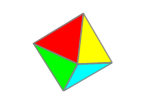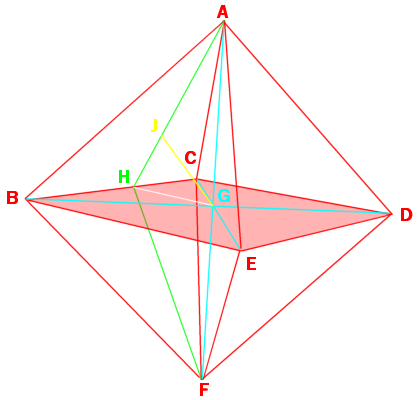# OctahedronThe octahedron is a Platonic solid, which means it is made of all regular polygons for each face, being eight equilateral triangles arranged four at each vertex.

With edge length of S, the surface area would be that of 8 equilateral triangles, $$8\cdot S^2\cdot \frac{\sqrt3}{4}=2\cdot S^2\sqrt3$$.

If we slice one in half, through 4 co-planar vertices, we get two 4-sided pyramids. Since we know that all the edges are the same length and all the angles are the same, the only possible shape for the base would have to be a square.

If there were only three triangles meeting at the apex, instead of four, we could use the formula found earlier to get the vertex edge angle, but we don’t need to recalculate a new formula. Slicing through any co-planar vertices will result in a square-based pyramid. Therefore, at each vertex, each edge is 60° to the ones on either side of it and 90° to the fourth.

Given an octahedron with edge length of 1 and vertices A-F, we can draw diagonals (AF, BD, CE) across opposite corners, each being the same length $$\sqrt2$$, and meeting at point G, the center of the octahedron. Half of these lengths is $$\frac{\sqrt2}{2} \text{, or } \frac{1}{\sqrt2}$$, which is the radius of the circumscribed sphere.

The midpoint of line BC is H. Line AH and FH are length $$\frac{\sqrt3}{2}$$. We now have a triangle AHF. We then can use the law of cosines to find ∠AHF,

\begin{align}\cos\angle AHF &=\frac{(AH)^2+(FH)^2-(AF)^2}{2(AH)(AF)}\\ &=\frac{\left(\frac{\sqrt3}{2}\right)^2+\left(\frac{\sqrt3}{2}\right)^2 – \sqrt2^2}{2\cdot\frac{\sqrt3}{2}\cdot\frac{\sqrt3}{2}} \\ &=\frac{\frac34+\frac34-2}{\frac32}\\ &=\left(\frac64-\frac84\right)\cdot\frac23 \\ &= -\frac24\cdot\frac23 \\ &= -\frac{4}{12}\\ &=-\frac13\end{align}

So $$\cos\angle AHF =-\frac13$$, ∠AHF= 109.4712206…°, the dihedral angle.

Placing point J at the circumcenter of ΔABC, gives a line AJ of length $$\sqrt{\frac13}$$, and ∠AJG is 90°. Solving for GJ gives:

\begin{align}(GJ)^2+(AJ)^2&=(AG)^2\GJ)^2 + \left(\frac{1}{\sqrt3}\right)^2 &= \left(\frac{1}{\sqrt2}\right)^2\\(GJ)^2 &= \left(\frac{1}{\sqrt2}\right)^2-\left(\frac{1}{\sqrt3}\right)^2\\(GJ)^2 &= \frac12-\frac13\\ &=\frac16 \end{align} \(GJ=\sqrt\frac16, the radius of an inscribed sphere.

Joining the centers of each triangle, gives the edges a cube, the dual of the octahedron.